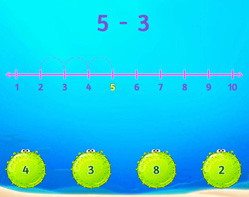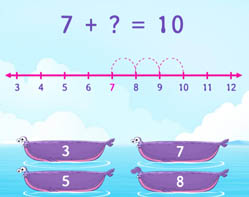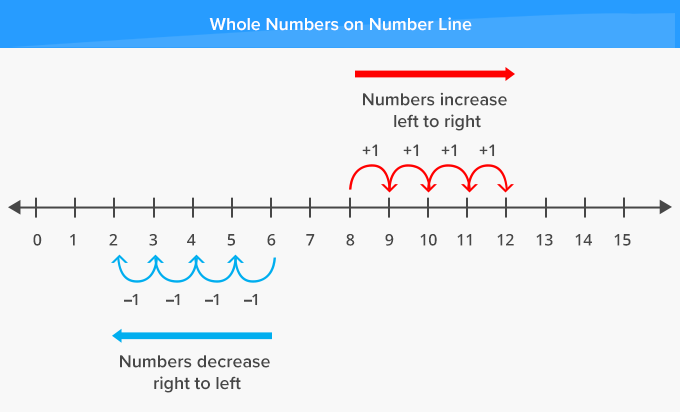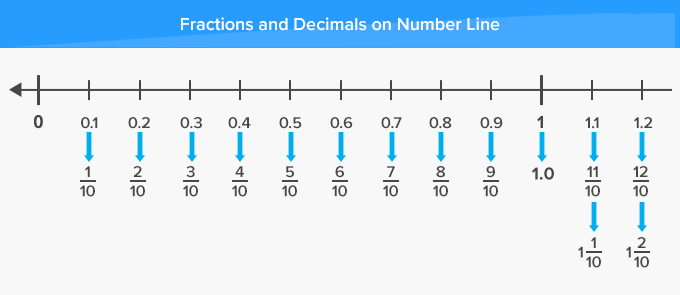# Number Line - Definition with Examples

The Complete K-5 Math Learning Program Built for Your Child

• 30 Million Kids

Loved by kids and parent worldwide

• 50,000 Schools

Trusted by teachers across schools

• Comprehensive Curriculum

Aligned to Common Core

## Number Line GamesCount Back to Subtract

Use the count back method to subtract. For example, to subtract 2 from 4, one can start at 4 and count back 2 steps.

Covers Common Core Curriculum 1.OA.5Count on to Add within 20

Count on to add numbers with sum within 20. Relate addition to counting using number lines, pictures and counting mentally.

Covers Common Core Curriculum 1.OA.5

## What is Number Line?

In math, a number line can be defined as a straight line with numbers placed at equal intervals or segments along its length.
A number line can be extended infinitely in any direction and is usually represented horizontally.

The numbers on the number line increase as one moves from left to right and decrease on moving from right to left.Here, fractions and decimals have been represented on the number line.A number line can also be used to represent both positive and negative integers as well.

Writing numbers on a number line makes comparing numbers easier. Numbers on the left are smaller than the numbers on the right of the number line.

A number line can also be used to carry out addition, subtraction and multiplication. We always move right to add, move left to subtract and skip count to multiply

 Fun Facts A blank number line was originally proposed as a visual model or diagram for solving addition and subtraction operations. An empty or blank number line is a visual diagram of a number line with no numbers or markers and is essentially used for solving word problems.

##Let's sing!

When comparing numbers, bigger than nine,
Write them on intervals of a number line.
Don’t you worry! You would do just fine!
Hop to the right, for numbers greater than nine!

##Let's do it!

Instead of handing out regular number line worksheets to your children, take them outside. Draw a big number line using chalk in the driveway. Ask them to compare, add and subtract numbers you say, by jumping on the numbers.
You further ask them to extend the number line or change the values of each interval for greater numbers.

##Related math vocabulary

Won Numerous Awards & Honors